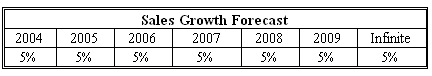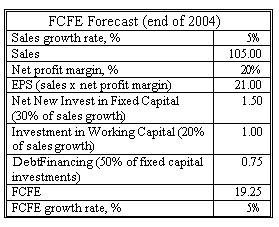### CFA Practice Question

There are 290 practice questions for this topic.

### CFA Practice Question

Uwe Henschel is doing a valuation of TechnoSchaft using the following information:

• 2003 sales per share = \$100.
• Net profit margin = 20%.
• Net new investment in fixed capital = 30% of sales increase (50% of these investments is financed with debt).
• Investment in working capital = 20% of sales increase.
• Beta = 1.3.
• Risk-free rate = 4%.
• Equity risk premium = 6%.

He forecasts the following future sales pattern for the company:The stock of the company is worth ______using the most appropriate type of FCFE model.
A. \$261
B. \$283
C. \$272
Explanation: The company is growing at a constant rate. We use the single stage model in this case.

First we calculate free cash flows to equity for the company:We then calculate the cost of equity, which will serve as the discount rate in our model: r = rf + beta x equity premium = 0.04 + 1.3 x 0.06 = 0.118, or 11.8%.

The value of the company can be calculated using the single-stage model: Equity value V2003 = FCFE2004 / (r - g) = 19.25 / (0.118 - 0.05) = \$283.